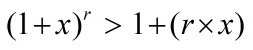Request a Tool

Online Bernoulli Inequality Calculator

Bernoulli Inequality Calculator use to calculate the bernoulli inequality of any real number with power.

Output

Bernoulli Inequality
0

Formula• x = Any real number
• r = It is the power value

Defination / Uses

Bernoulli's inequality is a mathematical inequality that approximates 1 + x exponentiations. It is frequently used in real-world analysis. For each integer r 0 and real number x 1, it has multiple useful variations.

An inequality that approximates exponentiations of 1 + x is known as Bernoulli's Inequality. For every integer r 0 and every real number x 1, the inequality asserts that The inequality holds for all real integers x if the exponent r is even. For every integer r 2 and every real number x 1 with x 0, the strict version of the inequality is that. By entering the values for x value and power increased to that value, this advanced online Bernoulli Inequality Calculator can determine the inequality of any given function.